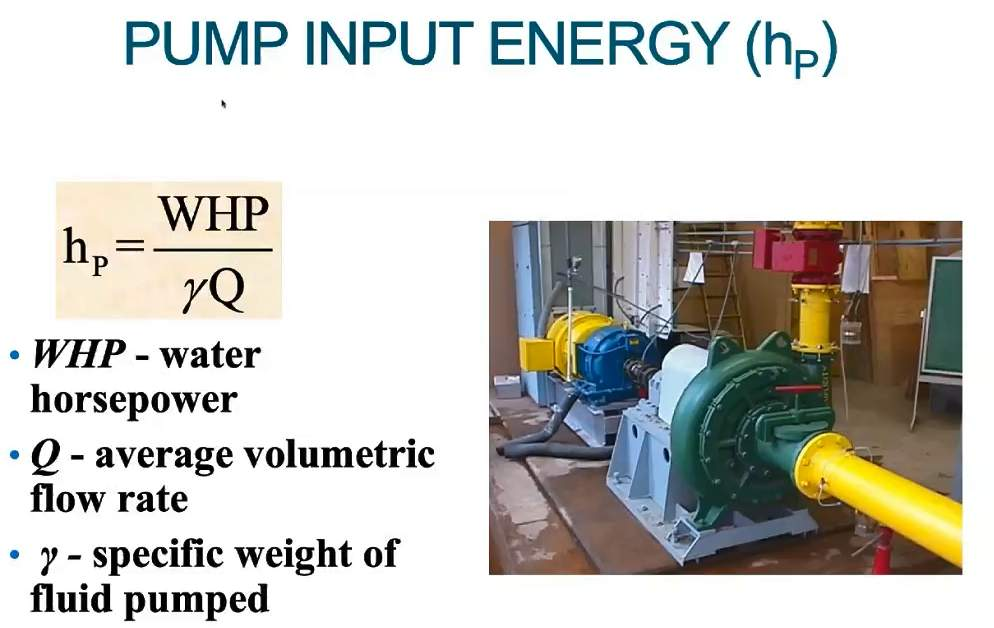# Fluid Mechanics Overview for Dredging

## Definitions of Fluid Mechanics Properties

• ρ = mass/volume = fluid density
• ρwater = 1.94 slugs/ft3 = 999 kg/3
• ρseawater = 1.99 slugs/ft3 = 1030 kg/m3
• g = length/time2 = acceleration due to gravity
• g = 32.2 ft/s2; 9.81 m/22
• y = weight/volume  = ρg = specific weight
• y water = 62.4 lb/ft3; 9.8 kN/m3
• y seawater = 64.0 lb/ft3; 10.1 kN/m3
• SG = specific gravity = ρsubstance /ρwater @ 4° Celcius
• SG SAE 30 = 0.91
• SG water = 1.00
• SG seawater = 1.03
• SG HG = 13.61
• SG slurry = 1.1 – 1.5
• SG (solid) sand = 2.65
• SG (loose) sand = 1.9 – 2.1
• μ – dynamic viscosity
• t = μ du/dy
• ⋎ – kinematic viscosity
• ⋎ = μ
• Re – Reynolds Number inertia force / viscous force
• Re = ρ D U/μ = U D/v    (pipe flow)
• U – average velocity in pipe
• D – pipe inside diameter

## Reynolds Number (Re) for Pipe Flow

To determine if slurry flow is laminar or turbulent

• Re < 2000    Laminar Flow
• Re > 4000   Turbulent Flow

Example:

Slurry (1.2 Specific Gravity) flows at 15 ft/2 through a 24 inch inside diameter pipe.

Is the flow laminar or turbulent?

Re = (1.94 slugs/ft3(1.25)(15 ft/s)(24 in/12 ft)2.34E-5 lb s/ft2)
Re = 3,110,000
Flow is TURBULENT

## Pressure Relationships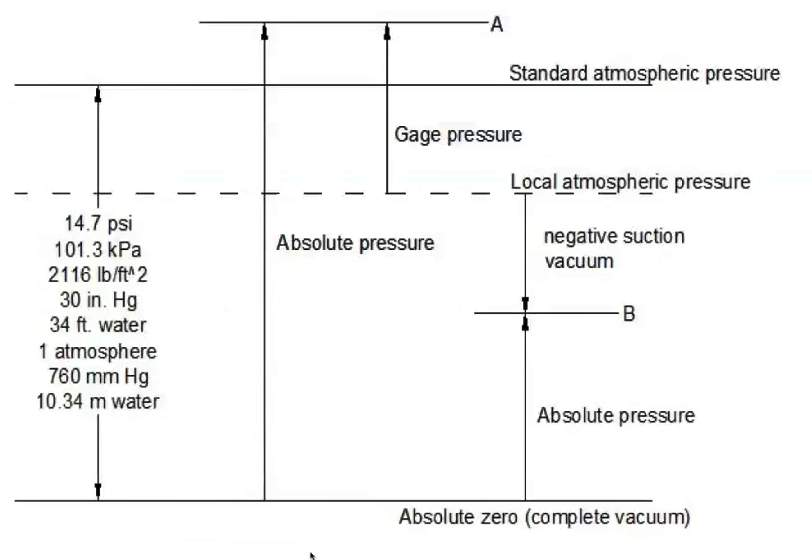## Pressure & Buoyancy

How pressure is quantified and buoyancy in individual sediment grains that are floating through your slurry. Buoyant force is equal to weight of the displaced fluid x by the specific weight of the displaced fluid.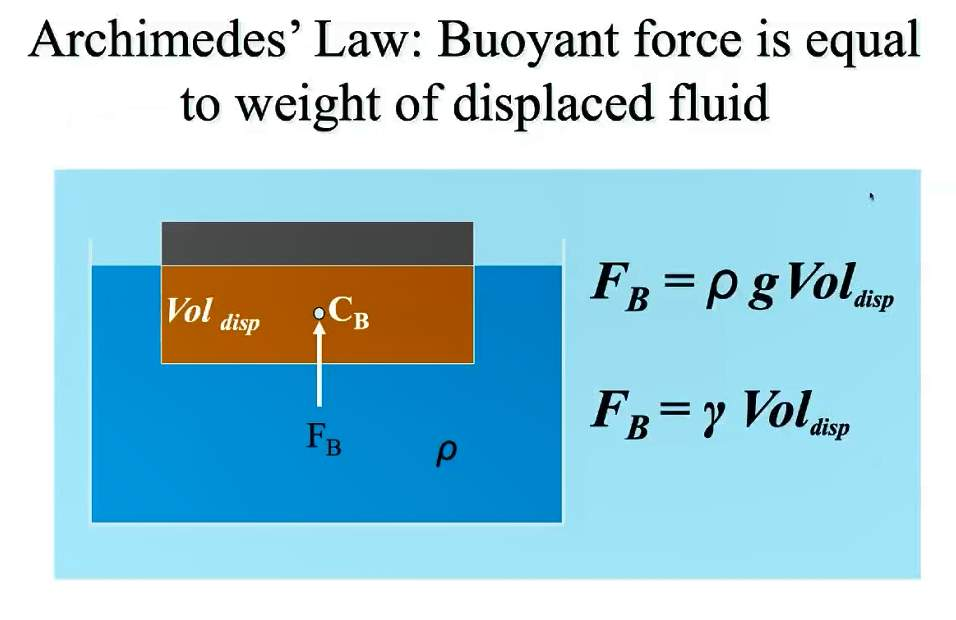## Continuity Equation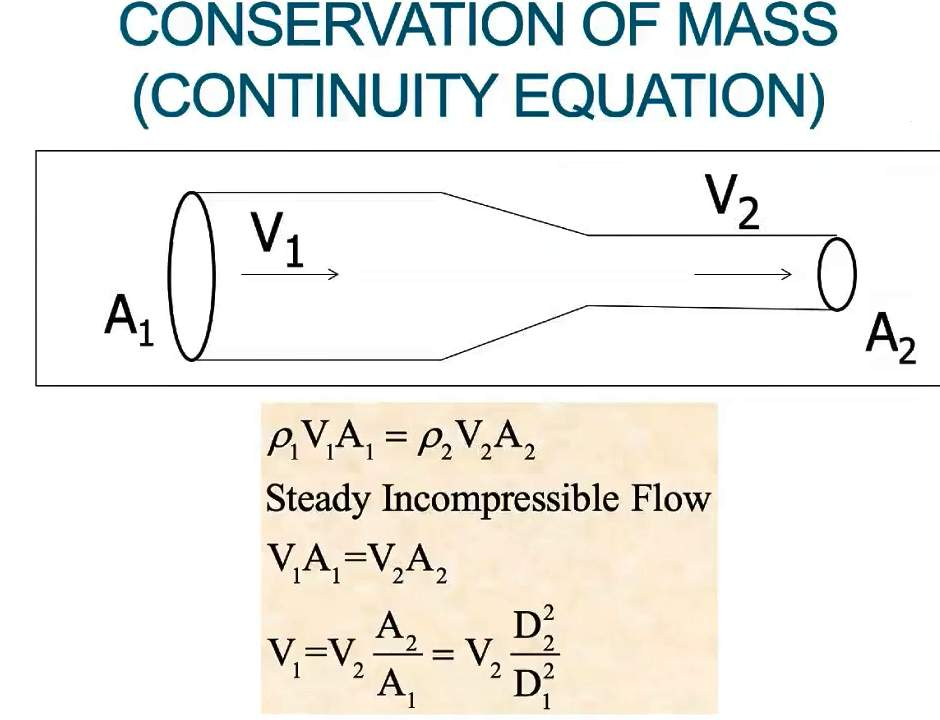## Bernoulli Equation

Compare different contributions to energy flow in pipe systems.  Calculate flow rates, velocities, losses.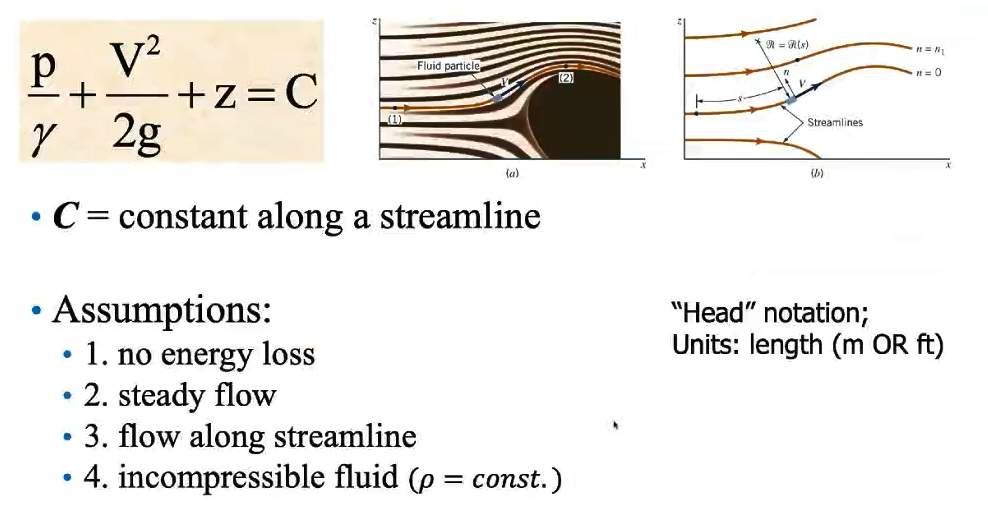## Energy Equation

Calculate Losses in Pipe Systems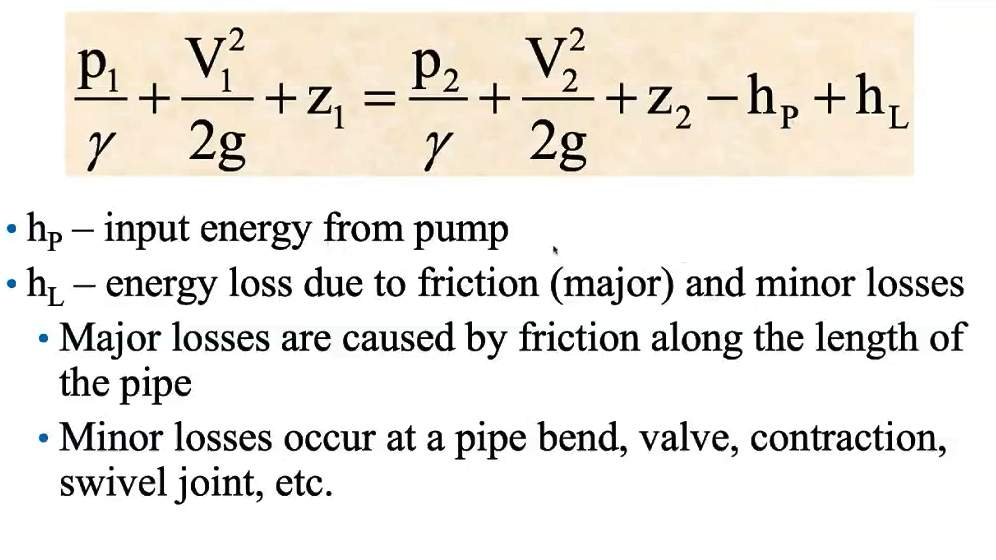## Friction Loss in Slurry Flow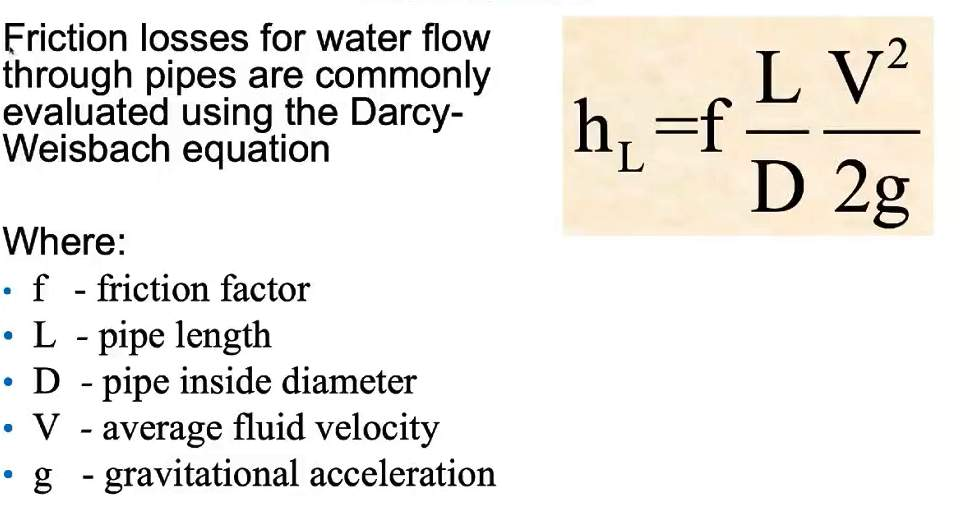## Moody Diagram – Friction in Slurry Flow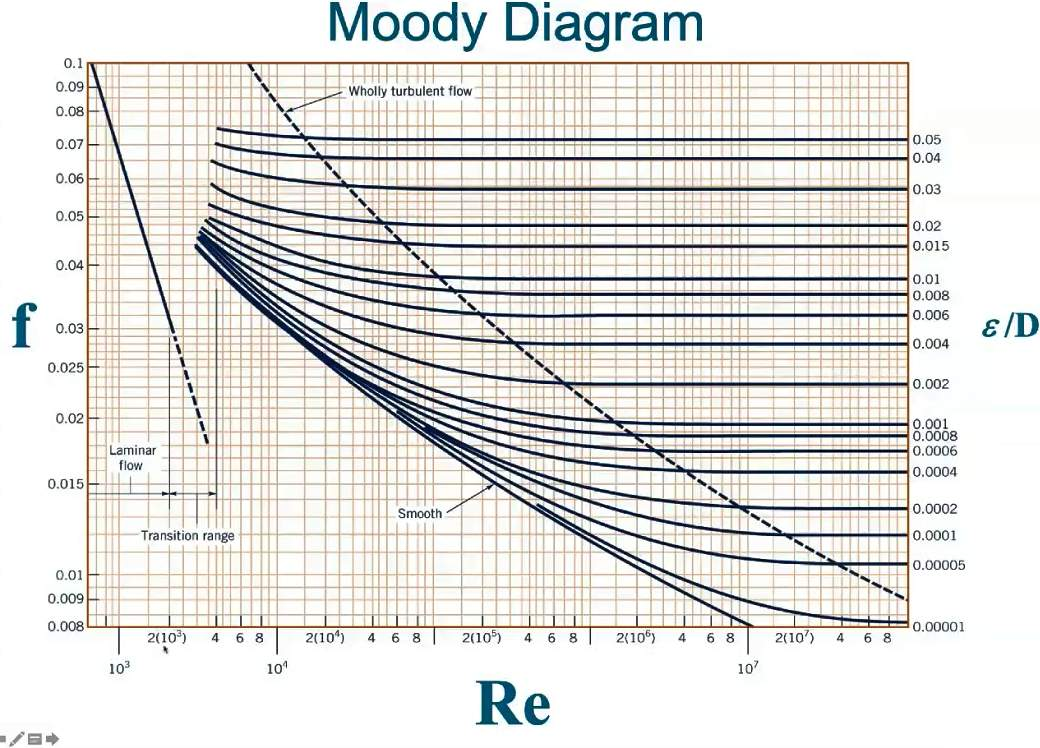## The equation for Calculating Friction Factor (f)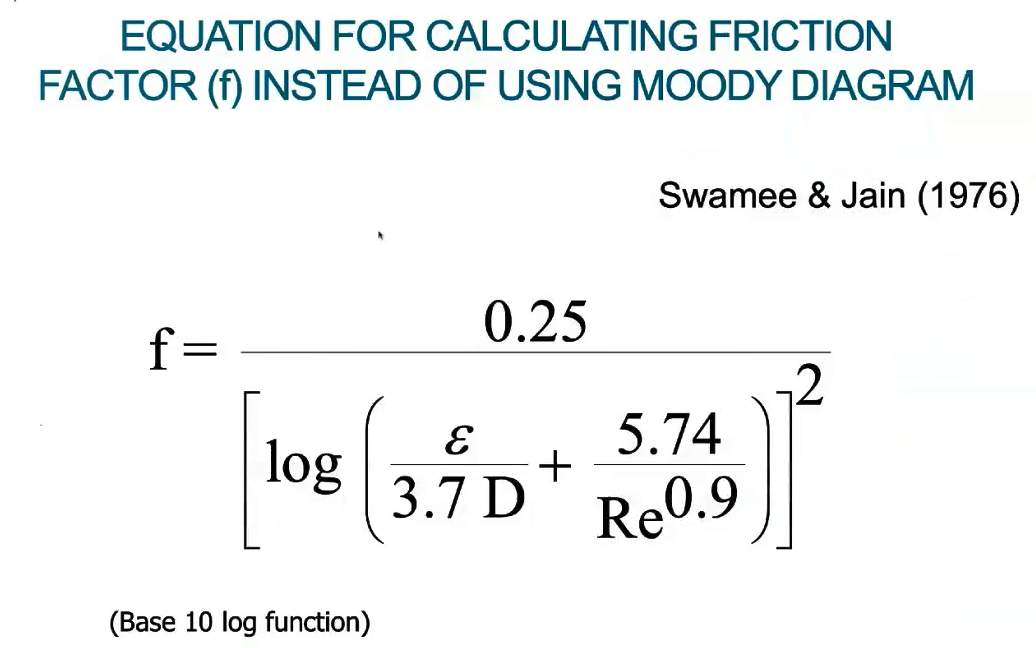## Relative Roughness of Slurry Pipelines Material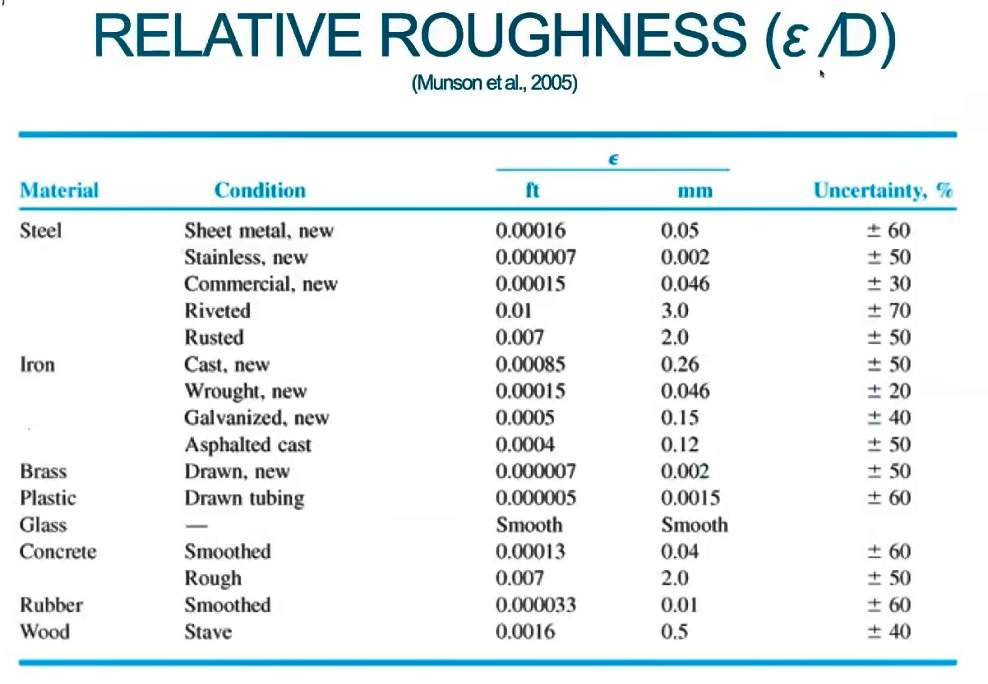## Equivalent Length of Pipe (losses)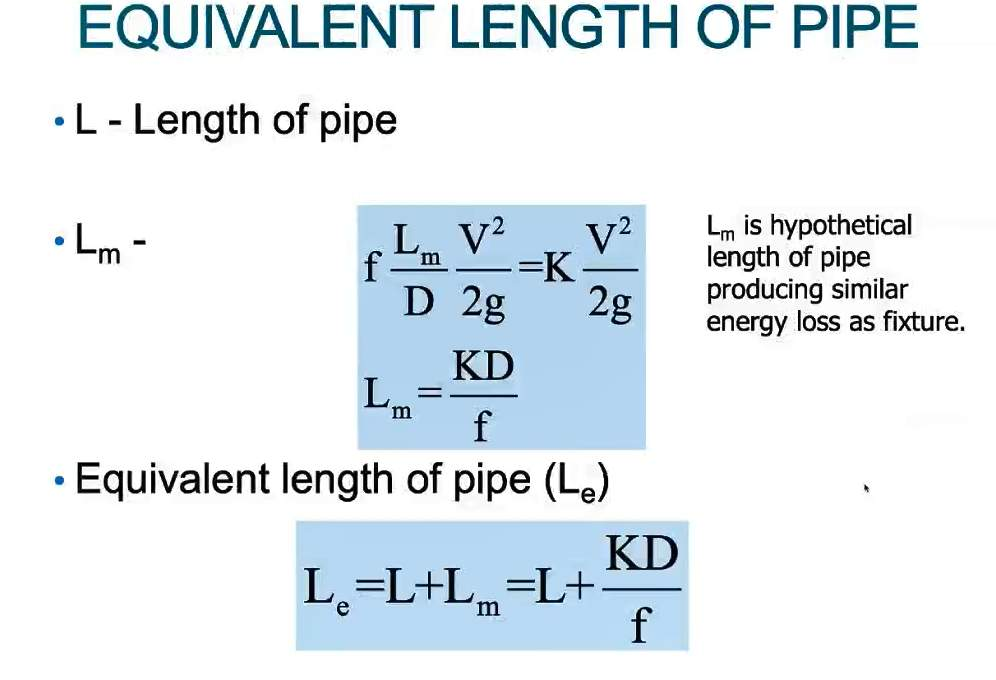## Pump Input Energy